Shortcuts

# Calibration Error¶

## Module Interface¶

class torchmetrics.CalibrationError(task: Optional[Literal['binary', 'multiclass']] = None, n_bins: int = 15, norm: Literal['l1', 'l2', 'max'] = 'l1', num_classes: = None, ignore_index: = None, validate_args: bool = True, **kwargs: Any)[source]

Top-label Calibration Error. The expected calibration error can be used to quantify how well a given model is calibrated e.g. how well the predicted output probabilities of the model matches the actual probabilities of the ground truth distribution.

Three different norms are implemented, each corresponding to variations on the calibration error metric.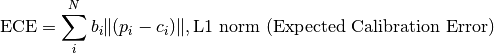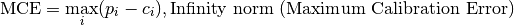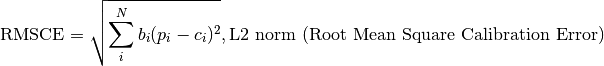Where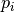is the top-1 prediction accuracy in bin,is the average confidence of predictions in bin, and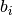is the fraction of data points in bin. Bins are constructed in an uniform way in the [0,1] range.

This function is a simple wrapper to get the task specific versions of this metric, which is done by setting the task argument to either 'binary' or 'multiclass'. See the documentation of BinaryCalibrationError and MulticlassCalibrationError for the specific details of each argument influence and examples.

### BinaryCalibrationError¶

class torchmetrics.classification.BinaryCalibrationError(n_bins=15, norm='l1', ignore_index=None, validate_args=True, **kwargs)[source]

Top-label Calibration Error for binary tasks. The expected calibration error can be used to quantify how well a given model is calibrated e.g. how well the predicted output probabilities of the model matches the actual probabilities of the ground truth distribution.

Three different norms are implemented, each corresponding to variations on the calibration error metric.Whereis the top-1 prediction accuracy in bin,is the average confidence of predictions in bin, andis the fraction of data points in bin. Bins are constructed in an uniform way in the [0,1] range.

As input to forward and update the metric accepts the following input:

• preds (Tensor): A float tensor of shape (N, ...) containing probabilities or logits for each observation. If preds has values outside [0,1] range we consider the input to be logits and will auto apply sigmoid per element.

• target (Tensor): An int tensor of shape (N, ...) containing ground truth labels, and therefore only contain {0,1} values (except if ignore_index is specified). The value 1 always encodes the positive class.

As output to forward and compute the metric returns the following output:

Additional dimension ... will be flattened into the batch dimension.

Parameters

Example

>>> from torchmetrics.classification import BinaryCalibrationError
>>> preds = torch.tensor([0.25, 0.25, 0.55, 0.75, 0.75])
>>> target = torch.tensor([0, 0, 1, 1, 1])
>>> metric = BinaryCalibrationError(n_bins=2, norm='l1')
>>> metric(preds, target)
tensor(0.2900)
>>> bce = BinaryCalibrationError(n_bins=2, norm='l2')
>>> bce(preds, target)
tensor(0.2918)
>>> bce = BinaryCalibrationError(n_bins=2, norm='max')
>>> bce(preds, target)
tensor(0.3167)


Initializes internal Module state, shared by both nn.Module and ScriptModule.

### MulticlassCalibrationError¶

class torchmetrics.classification.MulticlassCalibrationError(num_classes, n_bins=15, norm='l1', ignore_index=None, validate_args=True, **kwargs)[source]

Top-label Calibration Error for multiclass tasks. The expected calibration error can be used to quantify how well a given model is calibrated e.g. how well the predicted output probabilities of the model matches the actual probabilities of the ground truth distribution.

Three different norms are implemented, each corresponding to variations on the calibration error metric.Whereis the top-1 prediction accuracy in bin,is the average confidence of predictions in bin, andis the fraction of data points in bin. Bins are constructed in an uniform way in the [0,1] range.

As input to forward and update the metric accepts the following input:

• preds (Tensor): A float tensor of shape (N, C, ...) containing probabilities or logits for each observation. If preds has values outside [0,1] range we consider the input to be logits and will auto apply softmax per sample.

• target (Tensor): An int tensor of shape (N, ...) containing ground truth labels, and therefore only contain values in the [0, n_classes-1] range (except if ignore_index is specified).

Note

Additional dimension ... will be flattened into the batch dimension.

As output to forward and compute the metric returns the following output:

Parameters

Example

>>> from torchmetrics.classification import MulticlassCalibrationError
>>> preds = torch.tensor([[0.25, 0.20, 0.55],
...                       [0.55, 0.05, 0.40],
...                       [0.10, 0.30, 0.60],
...                       [0.90, 0.05, 0.05]])
>>> target = torch.tensor([0, 1, 2, 0])
>>> metric = MulticlassCalibrationError(num_classes=3, n_bins=3, norm='l1')
>>> metric(preds, target)
tensor(0.2000)
>>> mcce = MulticlassCalibrationError(num_classes=3, n_bins=3, norm='l2')
>>> mcce(preds, target)
tensor(0.2082)
>>> mcce = MulticlassCalibrationError(num_classes=3, n_bins=3, norm='max')
>>> mcce(preds, target)
tensor(0.2333)


Initializes internal Module state, shared by both nn.Module and ScriptModule.

## Functional Interface¶

torchmetrics.functional.calibration_error(preds, target, task=None, n_bins=15, norm='l1', num_classes=None, ignore_index=None, validate_args=True)[source]

Top-label Calibration Error. The expected calibration error can be used to quantify how well a given model is calibrated e.g. how well the predicted output probabilities of the model matches the actual probabilities of the ground truth distribution.

Three different norms are implemented, each corresponding to variations on the calibration error metric.Whereis the top-1 prediction accuracy in bin,is the average confidence of predictions in bin, andis the fraction of data points in bin. Bins are constructed in an uniform way in the [0,1] range.

This function is a simple wrapper to get the task specific versions of this metric, which is done by setting the task argument to either 'binary' or 'multiclass'. See the documentation of binary_calibration_error() and multiclass_calibration_error() for the specific details of each argument influence and examples.

Return type

Tensor

### binary_calibration_error¶

torchmetrics.functional.classification.binary_calibration_error(preds, target, n_bins=15, norm='l1', ignore_index=None, validate_args=True)[source]

Top-label Calibration Error for binary tasks. The expected calibration error can be used to quantify how well a given model is calibrated e.g. how well the predicted output probabilities of the model matches the actual probabilities of the ground truth distribution.

Three different norms are implemented, each corresponding to variations on the calibration error metric.Whereis the top-1 prediction accuracy in bin,is the average confidence of predictions in bin, andis the fraction of data points in bin. Bins are constructed in an uniform way in the [0,1] range.

Accepts the following input tensors:

• preds (float tensor): (N, ...). Preds should be a tensor containing probabilities or logits for each observation. If preds has values outside [0,1] range we consider the input to be logits and will auto apply sigmoid per element.

• target (int tensor): (N, ...). Target should be a tensor containing ground truth labels, and therefore only contain {0,1} values (except if ignore_index is specified). The value 1 always encodes the positive class.

Additional dimension ... will be flattened into the batch dimension.

Parameters

Example

>>> from torchmetrics.functional.classification import binary_calibration_error
>>> preds = torch.tensor([0.25, 0.25, 0.55, 0.75, 0.75])
>>> target = torch.tensor([0, 0, 1, 1, 1])
>>> binary_calibration_error(preds, target, n_bins=2, norm='l1')
tensor(0.2900)
>>> binary_calibration_error(preds, target, n_bins=2, norm='l2')
tensor(0.2918)
>>> binary_calibration_error(preds, target, n_bins=2, norm='max')
tensor(0.3167)

Return type

Tensor

### multiclass_calibration_error¶

torchmetrics.functional.classification.multiclass_calibration_error(preds, target, num_classes, n_bins=15, norm='l1', ignore_index=None, validate_args=True)[source]

Top-label Calibration Error for multiclass tasks. The expected calibration error can be used to quantify how well a given model is calibrated e.g. how well the predicted output probabilities of the model matches the actual probabilities of the ground truth distribution.

Three different norms are implemented, each corresponding to variations on the calibration error metric.Whereis the top-1 prediction accuracy in bin,is the average confidence of predictions in bin, andis the fraction of data points in bin. Bins are constructed in an uniform way in the [0,1] range.

Accepts the following input tensors:

• preds (float tensor): (N, C, ...). Preds should be a tensor containing probabilities or logits for each observation. If preds has values outside [0,1] range we consider the input to be logits and will auto apply softmax per sample.

• target (int tensor): (N, ...). Target should be a tensor containing ground truth labels, and therefore only contain values in the [0, n_classes-1] range (except if ignore_index is specified).

Additional dimension ... will be flattened into the batch dimension.

Parameters

Example

>>> from torchmetrics.functional.classification import multiclass_calibration_error
>>> preds = torch.tensor([[0.25, 0.20, 0.55],
...                       [0.55, 0.05, 0.40],
...                       [0.10, 0.30, 0.60],
...                       [0.90, 0.05, 0.05]])
>>> target = torch.tensor([0, 1, 2, 0])
>>> multiclass_calibration_error(preds, target, num_classes=3, n_bins=3, norm='l1')
tensor(0.2000)
>>> multiclass_calibration_error(preds, target, num_classes=3, n_bins=3, norm='l2')
tensor(0.2082)
>>> multiclass_calibration_error(preds, target, num_classes=3, n_bins=3, norm='max')
tensor(0.2333)

Return type

Tensor

© Copyright Copyright (c) 2020-2023, Lightning-AI et al... Revision 6853c553.

Built with Sphinx using a theme provided by Read the Docs.
Versions
latest
stable
v0.11.2
v0.11.1
v0.11.0
v0.10.3
v0.10.2
v0.10.1
v0.10.0
v0.9.3
v0.9.2
v0.9.1
v0.9.0
v0.8.2
v0.8.1
v0.8.0
v0.7.3
v0.7.2
v0.7.1
v0.7.0
v0.6.2
v0.6.1
v0.6.0
v0.5.1
v0.5.0
v0.4.1
v0.4.0
v0.3.2
v0.3.1
v0.3.0
v0.2.0
v0.1.0